# HBSE 7th Class Maths Solutions Chapter 6 The Triangles and Its Properties Ex 6.4

Haryana State Board HBSE 7th Class Maths Solutions Chapter 6 The Triangles and Its Properties Ex 6.4 Textbook Exercise Questions and Answers.

## Haryana Board 7th Class Maths Solutions Chapter 6 The Triangles and Its Properties Exercise 6.4

Question 1.
Is it possible to have a triangle with the following sides.
(i) 2 cm, 3 cm, 5 cm
(ii) 3 cm, 6 cm, 7 cm
(iii) 6 cm, 3 cm, 2 cm
Solution:
(i) 2 cm, 3 cm, 5 cm
∵ 2 cm + 3 cm = 5 cm
and third side = 5 cm
∴ Sum of the measure of lengths of two sides = length of third side which is not possible.
∴ A triangle cannot be possible with these sides.

(ii) 3 cm, 6 cm, 7 cm
∵ 3 cm + 6 cm = 9 cm and 9 cm > 7 cm
3 cm + 7 cm = 10 cm and 10 cm > 6 cm
6 cm + 7 cm = 13 cm and 13 cm > 3 cm
Thus, a triangle can be possible with these sides.(iii) 6 cm, 3 cm, 2 cm
∵ 6 cm + 3 cm = 9 cm and 9 cm > 2 cm
3 cm + 2 cm = 5 cm and 5 cm < 6 cm
2 cm + 6 cm = 8 cm and 8 cm > 5 cm
∴ A triangle cannot be possible with these sides.

Question 2.
Take any point O in the interior of a triangle PQR. Is
(i) OP + PQ > OQ ?
(ii) OQ + OR > QR ?
(iii) OR + OP > RP ?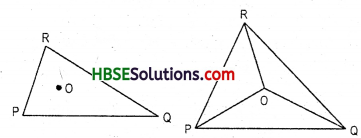Solution:
Sum of the lengths of any two sides of a triangle is greater than the length of than the length of the third side. (Fig. 6.47)
(i) Yes (ii) Yes (iii) Yes

Question 3.
AM is a median of a triangle ABC. Is AB + BC + CA + > 2 AM ?
Solution:
Since the sum of the lengths of any two sides of a triangle is greater than the length of the third side.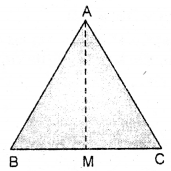∴ In ΔABM, we have AB + BM > AM ..,(i)
Similarly in AACM, CA + CM > AM …(ii)
Adding (i) and (ii), we have (AB + BM) + (CA + CM) > AM + AM
⇒ [AB + (BM + CM) + CA] > 2AM
⇒ [AB + BC + CA] > 2AM

Question 4.
ΔBCD is quadrialteral. Is AB + BC + CD + DA > AC + BD ?
Solution:
In ΔABC, AB + BC > AC …(i)
Sum of the lengths of any two sides of a triangle is greater than the length of the third side.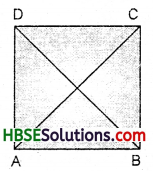In ΔACD, CD + DA > AC …(ii)
Adding (i) and (ii), we have
AB + BC + CD + DA > AC + AC …(iii)
In ΔABD, AB + DA > BD …(to)
In ΔBCD, BC + CD > BD …(v)
Adding (iv) and (v), we get
AB + BC + CD + DA > BD + BD …(vi)
2 (AB + BC + CD + DA) > 2(AC + BD)
AB + BC + CD + DA > AC + BD.Question 5.
ABCD is quadrilateral. Is AB + BC + CD + DA + < 2 (AC + BD) ?
Solution:
In ΔOAB
OA + OB > AB …(i)
[Sum of the lengths of any two sides of a triangle is greater than the length of the third side]
In ΔOBC, OB + OC > BC …(ii)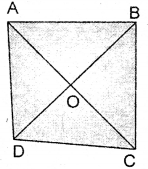In ΔOCD, OC + OD > DC …(Hi)
Adding (i), (ii), (iii) and (iv)
2(OA + OB + OC + OD) > AB + BC + CD + DA
⇒ AB + BC + CD + DA < 2(OA + OB + OC + OD)
⇒ AB + BC + CD + DA < 2(OA + OC + OB + OD)
AB + BC + CD + DA < 2(AC + BD).

Question 6.
The lengths of two sides of a triangle are 12 cm and 15 cm. Between what two measures should the length of the third side fall ?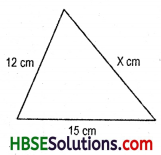Solution:
If x cm be the length of third side, we should have
12 + 15 > x ⇒ 27 > x
x + 12 > 15 ⇒ x > 3
and x + 15 > 12 ⇒ x > – 3
The numbers between 3 and 27 satisfy these.
∴ The length of the third side could be any length between 3 cm and 27 cm.